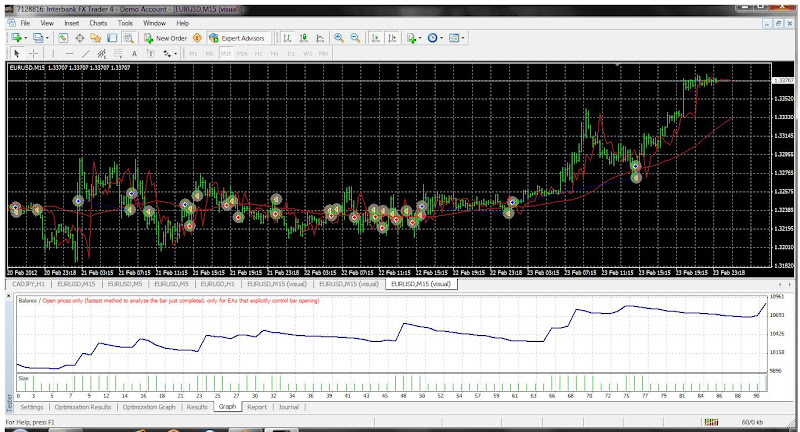## String equations boosting common strategies: Moving average with String equations

I got from mbkernel one important idea, that the neural net is a kind of a turbo charger. It  would help to squeeze more pips from already profitable strategy.

And the idea is that you have to start from something simple in the beginning.

For example the idea behind the fractal scalper is working without any "kernel tricks". "The kernel tricks" we deploy would help to squeeze more pips.

So that is why I consider those functions as an add-on to any good idea and not an end in themselves.

I was experimentig today with the simple Moving average EA available as the default EA in MT4. Everybody knows that  EA, the most basic of all EA, if the price closes above the Moving average Buy if it closes below the moving average Sell. Right?

In the default EA I was training from 16.02.12 to 24.02.12. And as you can imagine there were a lot of good results. But when I run the EA out of sample (from 25.02. 12 until today 21.02.12) most of those good settings did not perform (especially the most profitable). Several out of sample results were really good.

But how to know which one are good and which one are bad?

Despite all the efforts it still looks like gambling, which settings to take. On what settings to bet?

Is there anything that will tell you from the good settings which one are really good and which one are deceptive. You know this is a BIG question.

This is a central question after all for all trading algorythm.

Then to this basic strategy I applied a string equation, and I let the genetic optimizer choose the best lenght of the moving average.

I was very surprised it worked quite well.

And the first 10 results they all worked.

In the normal Moving average EA it produce also profitable in sample results but out of sample there is no way to know beforehand which settings to use some are proftable some not at all.

The string equation looks like this:

double stringMA()

{
double s1 = iMA(NULL,0,x1,MovingShift,MODE_LWMA,PRICE_CLOSE,0);// here we use
double s2 = iMA(NULL,0,x2,MovingShift,MODE_LWMA,PRICE_CLOSE,3);// delayed ma
double s3 = iMA(NULL,0,x3,MovingShift,MODE_LWMA,PRICE_CLOSE,5);
}

{
double re1=n/(2*L);
double re2=T/M;
double re3=MathSqrt(re2);
double re4=re1*re3;
}

{
int Length = MathFloor((re4 * x1 + re4 * x2 + re4*x3) / 3); // I want to have an integer
double p1 = iMA(NULL,0,Length,MovingShift,MODE_LWMA,PRICE_CLOSE,0);
return(p1);
}

}

The string parameters are:

extern double n=1;// nth harmonic optimize from 1 to 40 with step 1
extern double f=1;// frequency optimize from 1 to 40 with step 0.5
extern double T=1;//is the tension optimize from 1 to 40 with step 0.1
extern double M=1;is the linear density you do not have to optimize this and set to 1

extern int x1=1

extern int x2=1

extern int x3=1

With the genetic optimizer we are looking for the Lenght of the string.

And then ma=stringMA() that is how it is used in the expert

Yes I know about the classical string equation and the lenght of the indicator that is something new.whereis the tensionis the linear density, andis the length of the vibrating part of the string, and frequency, and nth harmonic .

• the shorter the string, the higher the frequency of the fundamental
• the higher the tension, the higher the frequency of the fundamental
• the lighter the string, the higher the frequency of the fundamental

It is realy fun to look at an indicator as a vibrating string and to map the indicator into a feature space and let the genetic optimizer to find the best settings.This is something I did not find elsewhere. So consider this as a total improvisation.

I was just imaging the indicator watching this picture on Wikipedia. So we plot the delayed indicator and we adapt using the string function that maps into a complex space. We let the optimizer to choose from the best settings. And for example if you see the output you see the suggested numbers. Actually you can compute manually and see what the suggested number really is.От Поле за пускане